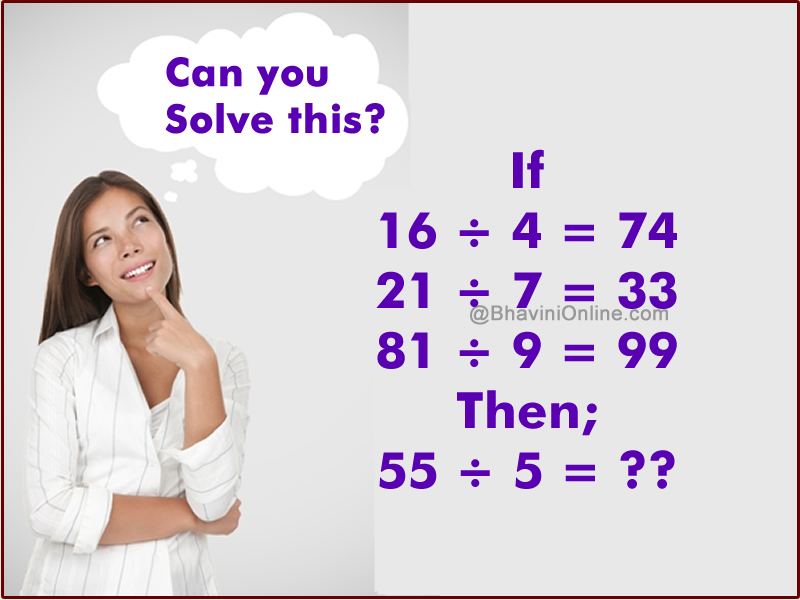# Math Riddle: If 16 ÷ 4 = 74 Then; 55 ÷ 5 = ??

Look at the equations and try to find the connection between the numbers.

In the last equation then find out the missing number.

If

16 ÷ 4 = 74

21 ÷ 7 = 33

81 ÷ 9 = 99

Then;

55 ÷ 5 = ??The numbers in the equations are of the form.

ab ÷ c = (a+b),(ab  ÷ c)

So in case of the first equation we have;

16 ÷ 4 = (1 + 6),(16  ÷ 4) = 74

Similarly we can try with the other 2 equations;

21 ÷ 7 = (2 + 1),(21 ÷7) = 33

&

81 ÷ 9 = (8 + 1),(81 ÷ 9) = 99

Hence we can find the missing number as;

55 ÷ 5 = (5 + 5),(55 ÷5) = 1011